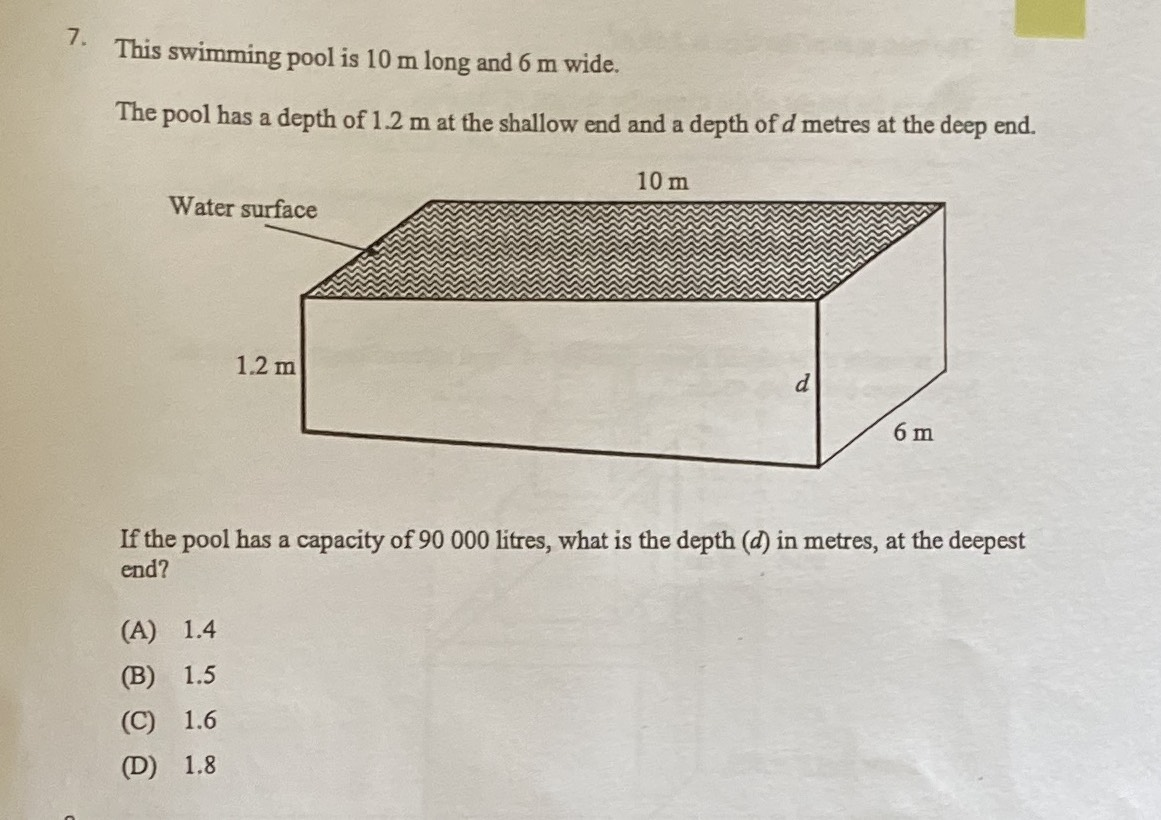### Still have math questions?

Algebra
QuestionThis swimming pool is $$10 m$$ long and $$6 m$$ wide. The pool has a depth of $$1.2 m$$ at the shallow end and a depth of $$d$$ metres at the deep end.

If the pool has a capacity of $$90000$$ litres, what is the depth $$( d )$$ in metres, at the deepest end? (A) $$1.4$$ (B) $$1.5$$ (C) $$1.6$$ (D) $$1.8$$